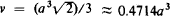# Regular Octahedron

Also found in: Dictionary, Thesaurus, Wikipedia.

## regular octahedron

[′reg·yə·lər ‚äk·tə′hē·drən]
(mathematics)
A regular polyhedron of eight faces.

## Octahedron, Regular

one of the five regular polyhedrons. An octahedron has eight faces (triangles), 12 edges, and six vertices; at each vertex four edges meet. If a is the length of an edge, then the octahedron’s volume is.

Site: Follow: Share:
Open / Close